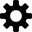聽力測驗 Numbers - Continued Whoops, we thought your browser supported HTML5 audio and it doesn't. Click here to report the issue.fractions
 1/2 1/3 1/4 1/5 2/3 3/4 3/2

a half dozen

I'll give you half.

 even number 偶數 odd number 奇數 whole number 整個數

 to count 算

My nephew knows how to count to ten.

 Arithmetic 算術

 to calculate 計算

What's the sum?
/
What's the total?

 value 值 average 平均

 Subtraction

10 - 4 = 6

Subtracting 4 from 10 gives you 6.

5 + 7 = 12

Adding 5 to 7 gives you 12.

 Multiplication

3 × 6 = 18

Multipling 3 by 6 is 18.
3由6的乘積是18。

 Division
 remainder 其餘

32 ÷ 8 = 4

Dividing 32 by 8 gives you 4.
8除以32，給你4。